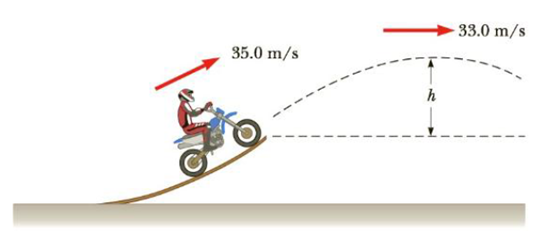Chapter 5, Problem 25P

Chapter
Section
Textbook Problem

A daredevil on a motorcycle leaves the end of a ramp with a speed of 35.0 m/s as in Figure P5.25. If his speed is 33.0 m/s when he reaches the peak of the path, what is the maximum height that he reaches? Ignore friction and air resistance.Figure P5.25

To determine
The maximum height that the daredevil reaches.

Explanation

Given Info:

The initial speed of the motorcycle when it leaves the ramp is 35.0m/s

The speed of the motor cycle when it reaches it maximum height is 33.0m/s .

Since, the friction and air resistance are ignored; only the gravitational force acts on the motorcycle and the rider in air.

So, according to conservation of energy,

12mvf2+mgxf=12mvi2+mgxi       (I)

• m is the mass of the system
• g is acceleration due to gravity
• vf is the final speed of the rider
• vi is the initial speed of the rider
• xf is the final height of the rider
• xi is the initial height of the rider

The maximum height that the rider reaches is,

h=xfxi

Hence, from equation (I), on re-arranging,

h=vi2vf22g

Substitute 35

Still sussing out bartleby?

Check out a sample textbook solution.

See a sample solution

The Solution to Your Study Problems

Bartleby provides explanations to thousands of textbook problems written by our experts, many with advanced degrees!

Get Started